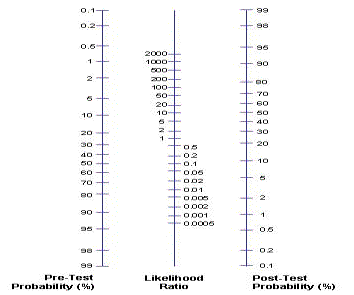# Pre- and post-test probability

Pre-test probability (~ prevalence)

This is the proportion of people in the population at risk who have the disease at a specific time or time interval, i.e. the point prevalence or the period prevalence of the disease. In other words, it is the probability − before the diagnostic test is performed − that a patient has the disease. Pre-test probabilities may be estimated from routine data, practice data or clinical judgement.

Post-test probability

This is the proportion of patients testing positive who truly have the disease. It is similar to the positive predictive value but apart from the test performance also includes a patient-based probability of having disease

 Post-test probability = Post-test odds (1 + Post-test odds)

Using pre- and post-test probability and LR

By comparing the pre- and post-test probabilities, it is possible to determine whether the probability of diagnosis has risen (i.e. the post-test probability has increased) or fallen (i.e. post-test probability has decreased). In this way, it is possible to provide comprehensive information about a screening test in order to enable informed choice. In practice assessing post-test probability is commonly done by using a Likelihood Ratio Nomogram (below):Summary of calculations of key features of screening tests

 Feature Formula Sensitivity a/ (a+c) Specificity d/ (b+d) Positive predictive value a/ (a+b) Negative predictive value d/ (c+d) Accuracy (a+d)/ (a+b+c+d) Likelihood ratio: Positive test Sensitivity/ (1-specificity) Negative test (1-sensitivity)/specificity

© Dr Murad Ruf and Dr Oliver Morgan 2008, Dr Kelly Mackenzie 2017